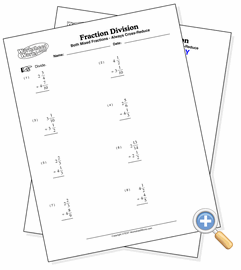# With Two Mixed Fractions

## Fraction DivisionDevelop division skills involving two mixed fractions

These problems involve the division of two mixed fractions. This provides the most difficult fraction multiplication problem since it involves converting to improper fractions, inverting the second fraction, cross-reducing, multiplying and finally simplifying in each problem.